## 匿名函数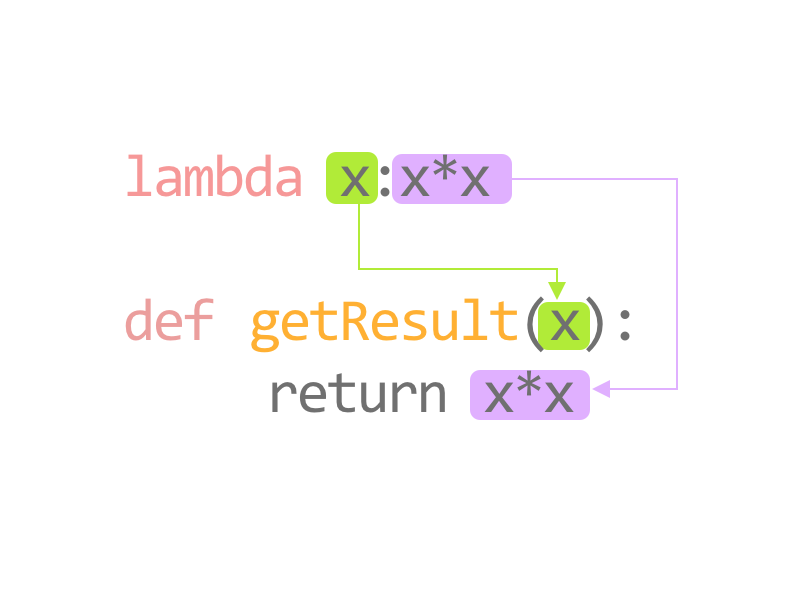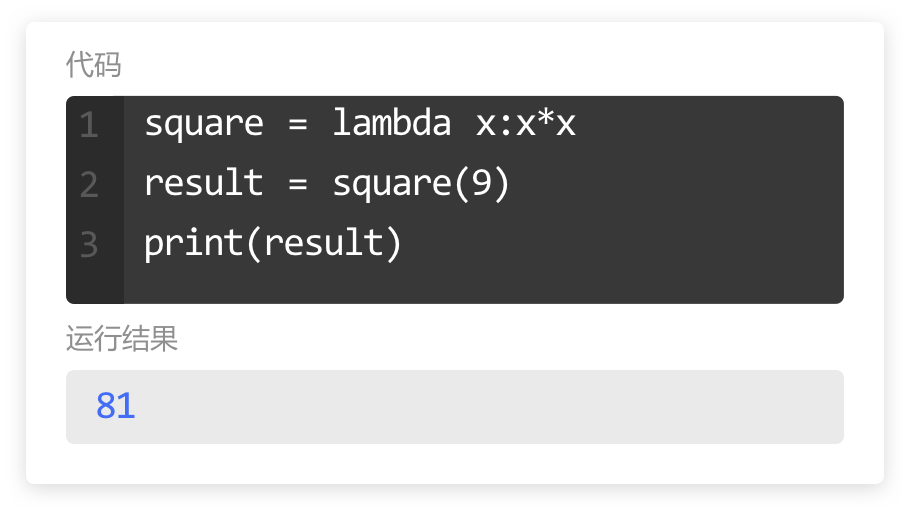### 匿名函数的结构

``````square = lambda x:x*x
result = square(9)
print(result)

multiply = lambda x,y:x*y
newResult = multiply(9,10)
print(newResult)``````

lambda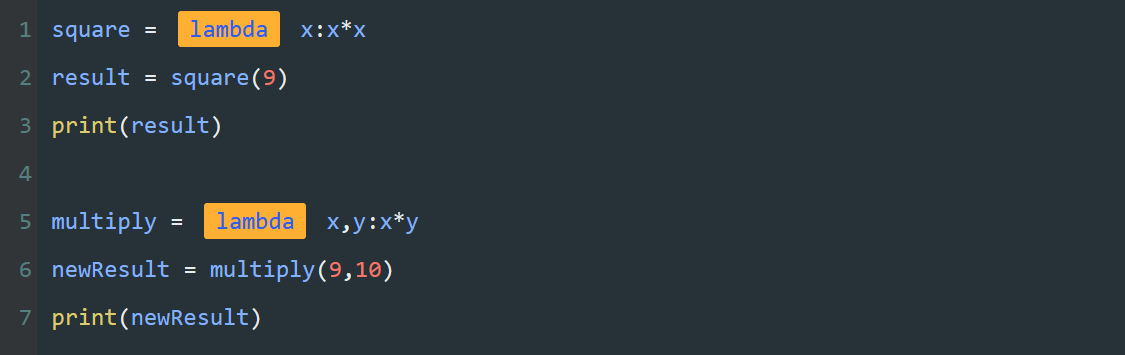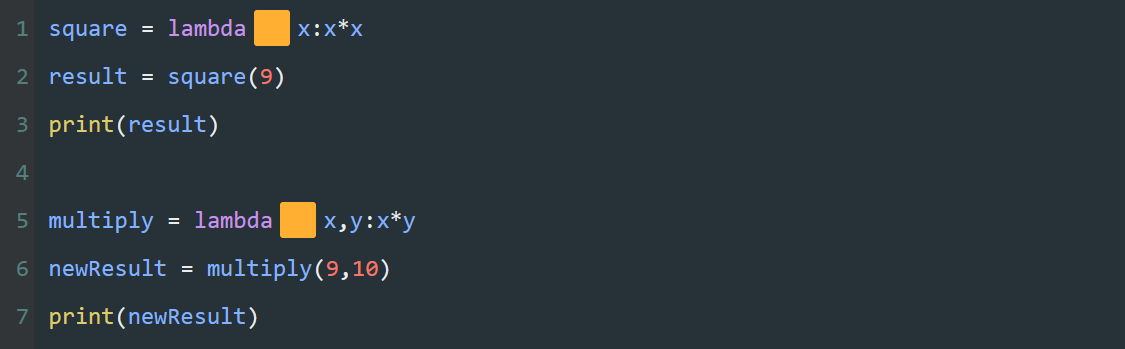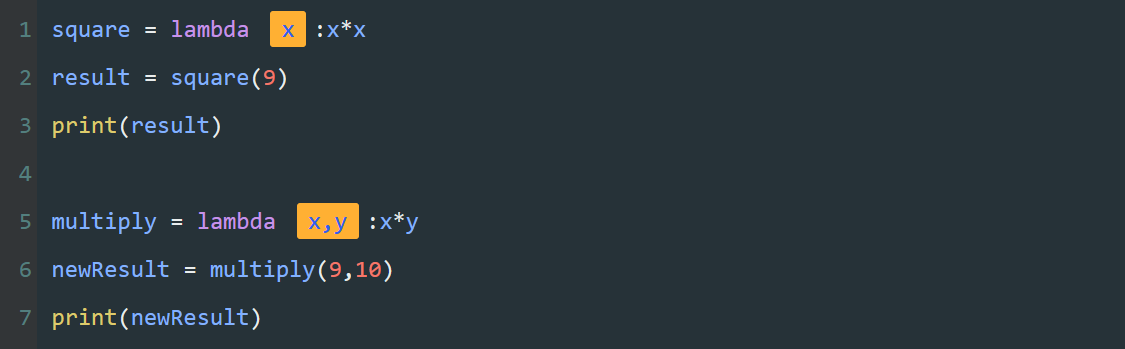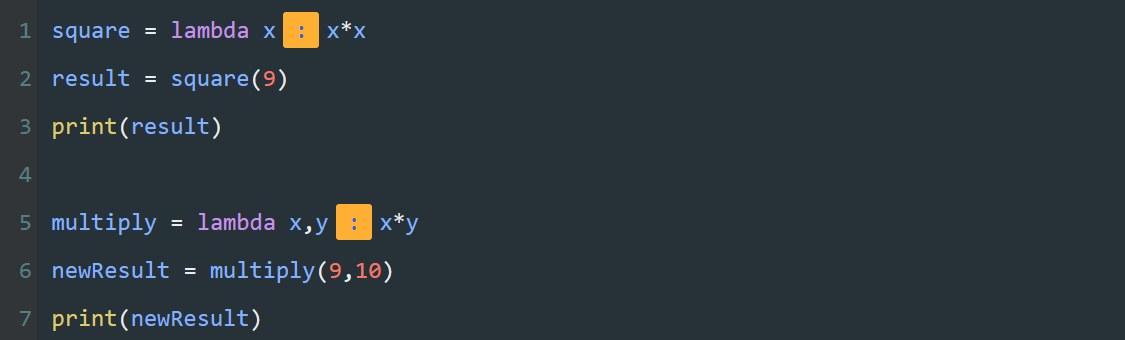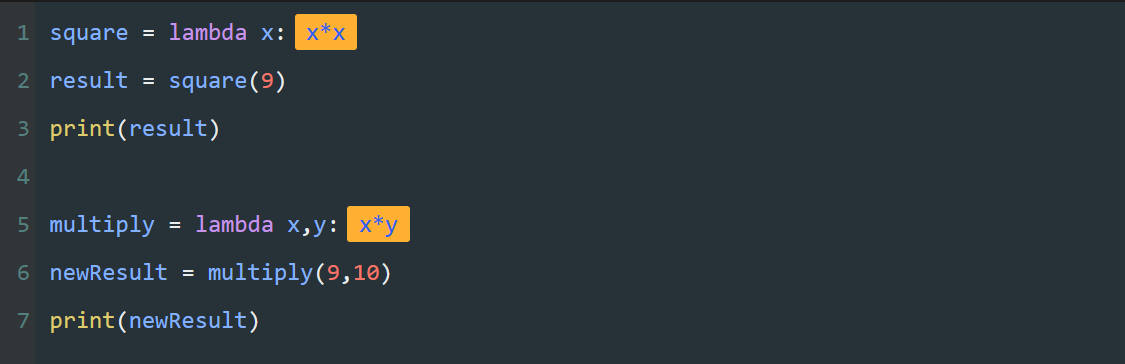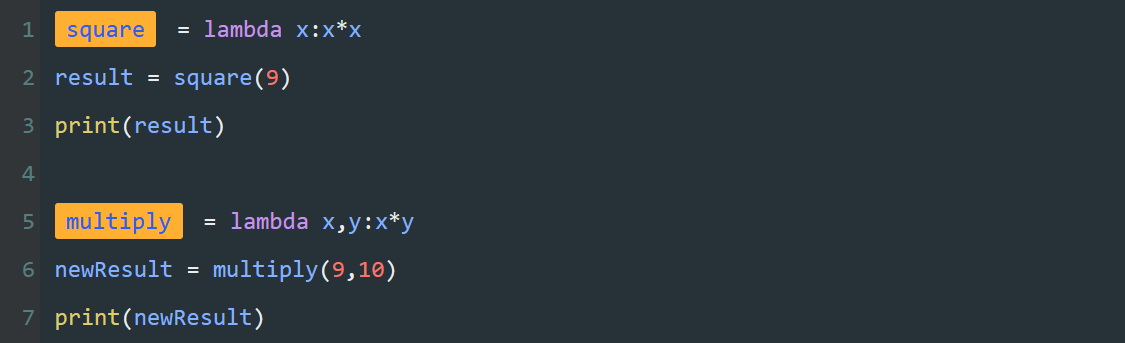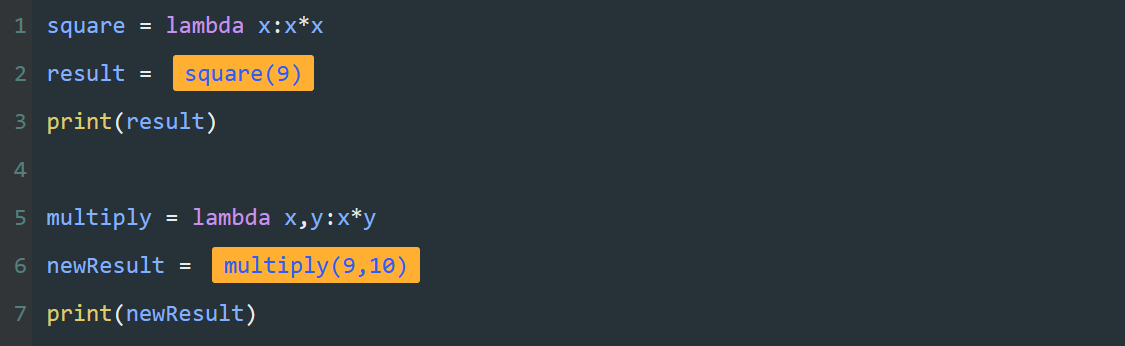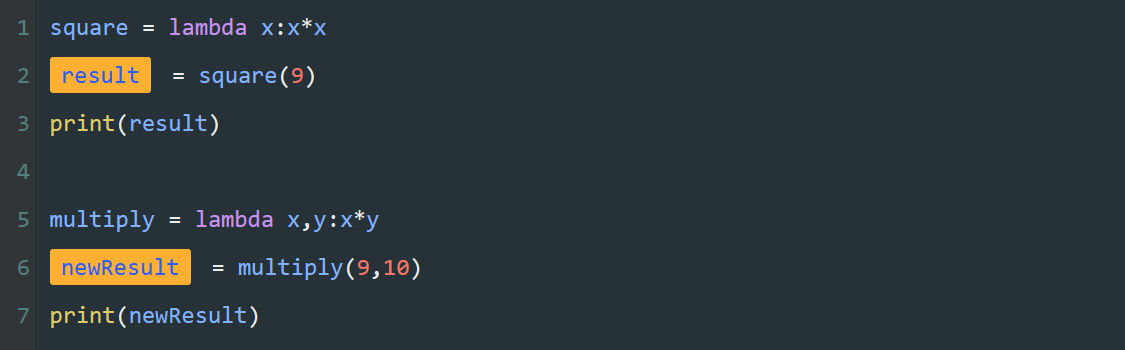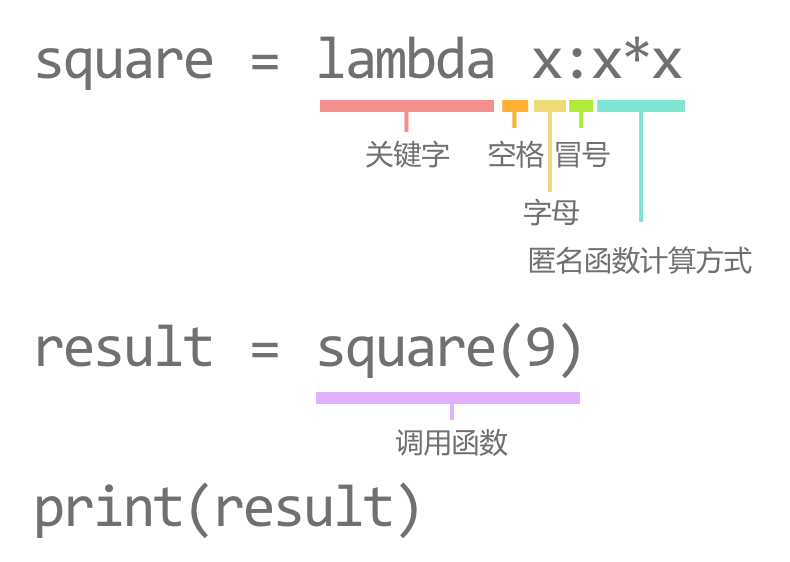### 小练习

``````power = lambda number:number*number*number
print(power(5))``````

``````area = lambda length,width,height:length*width*height

print(area(10, 8, 6))``````

`lambda` 的主体是一个表达式，而不是一个代码块，不适合处理复杂的逻辑情况。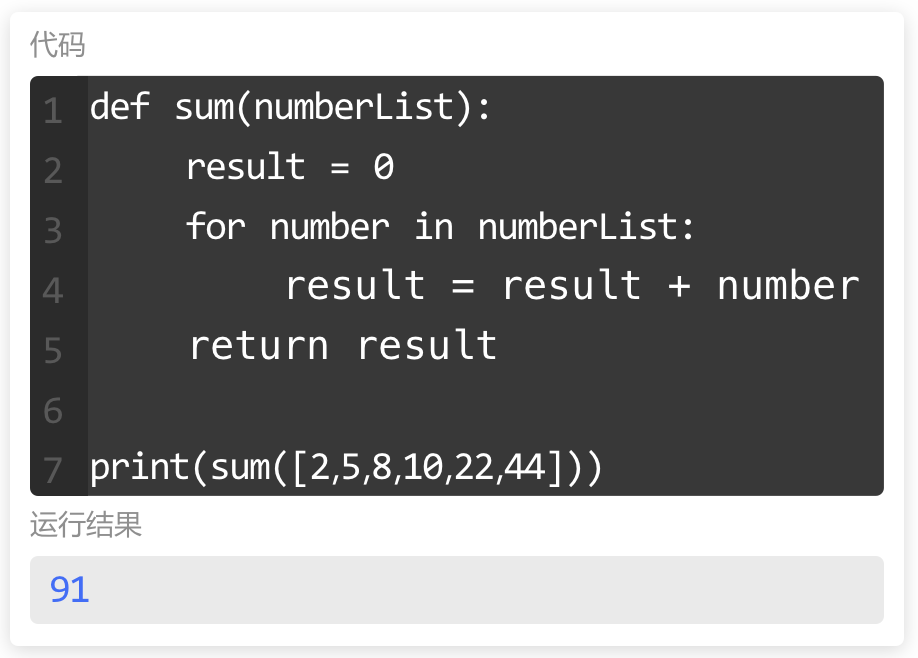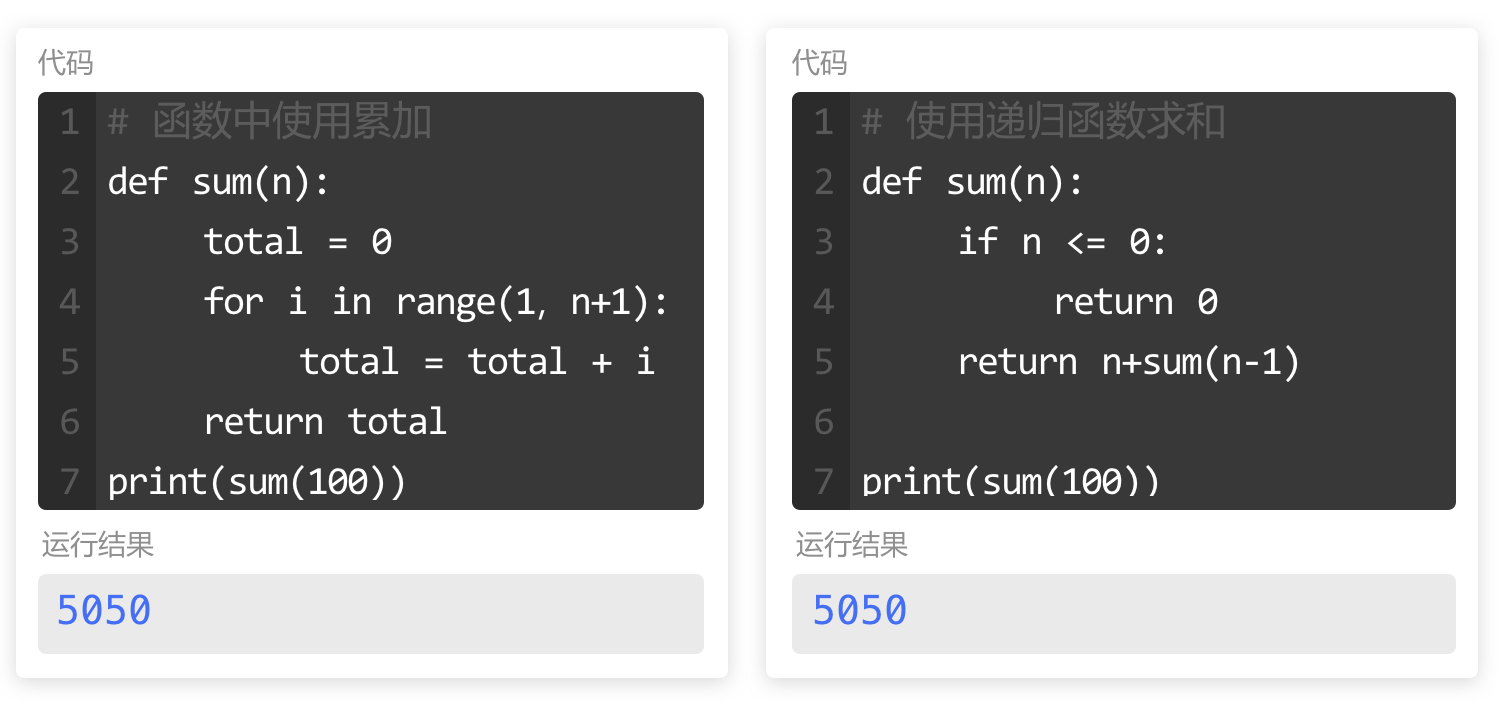## 递归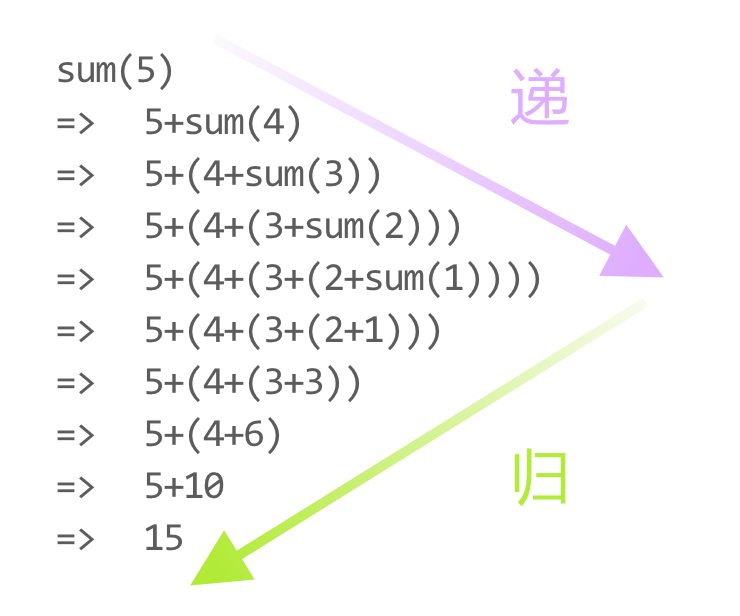## 递归

5+4+3+2+1+0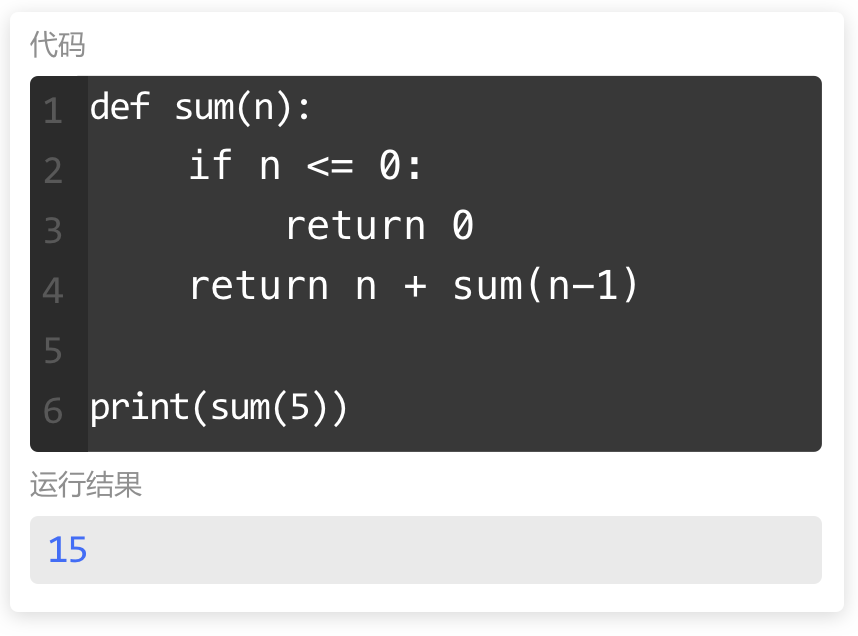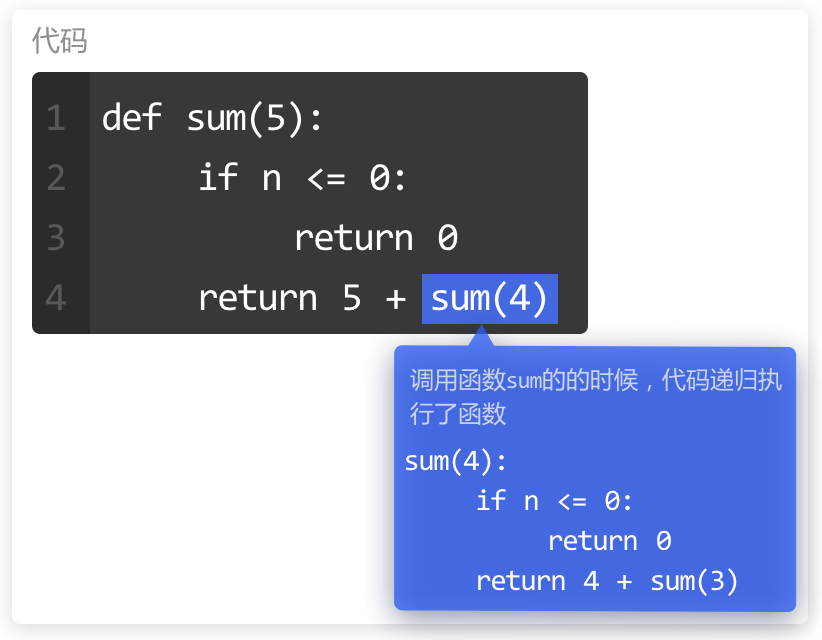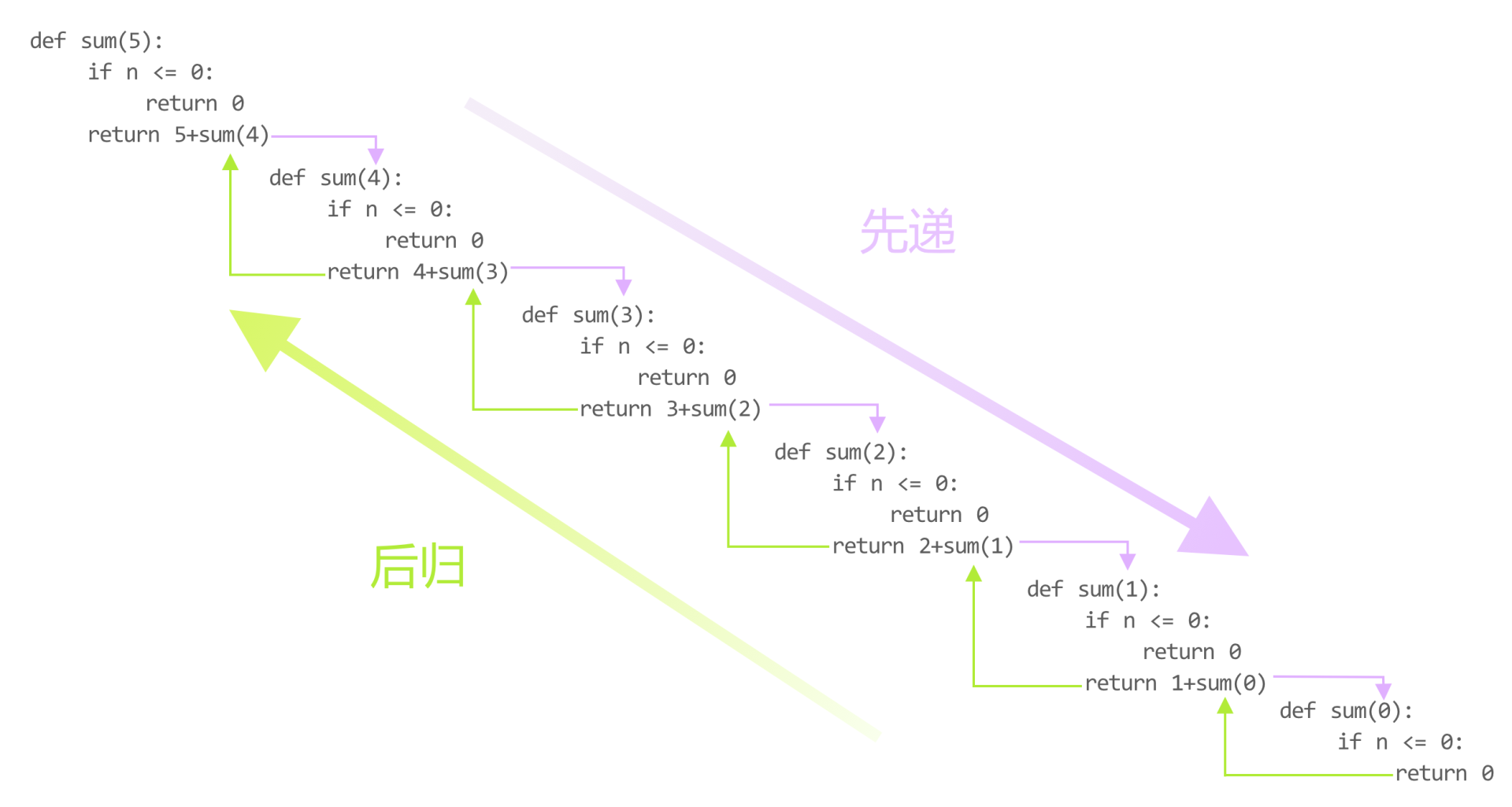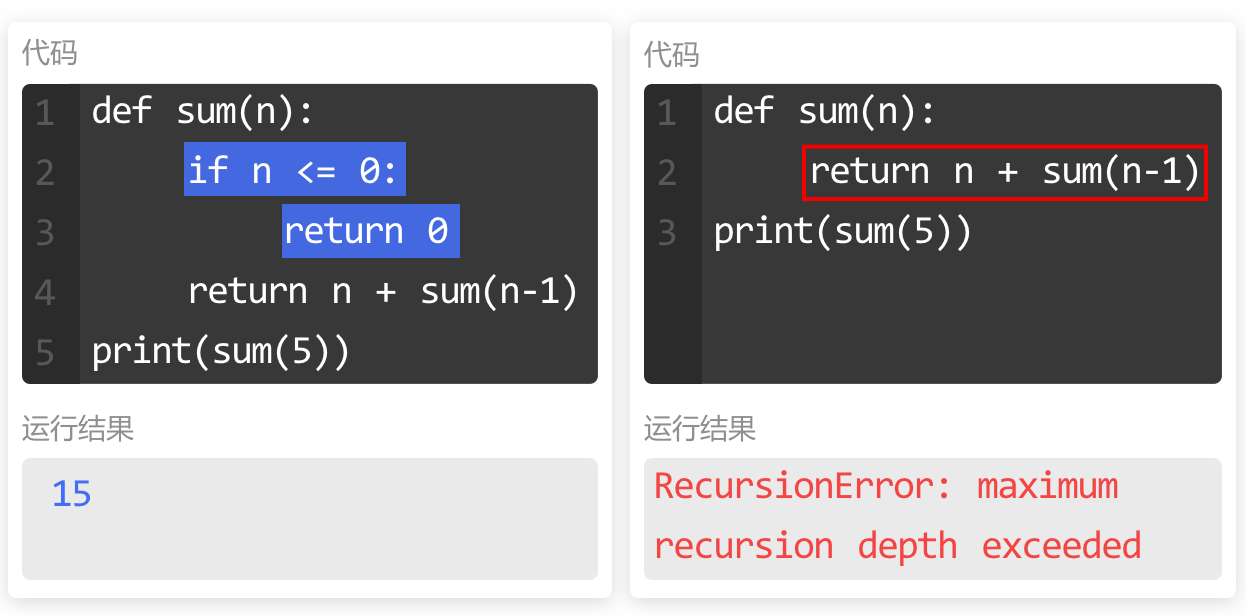`5! = 5×4×3×2×1`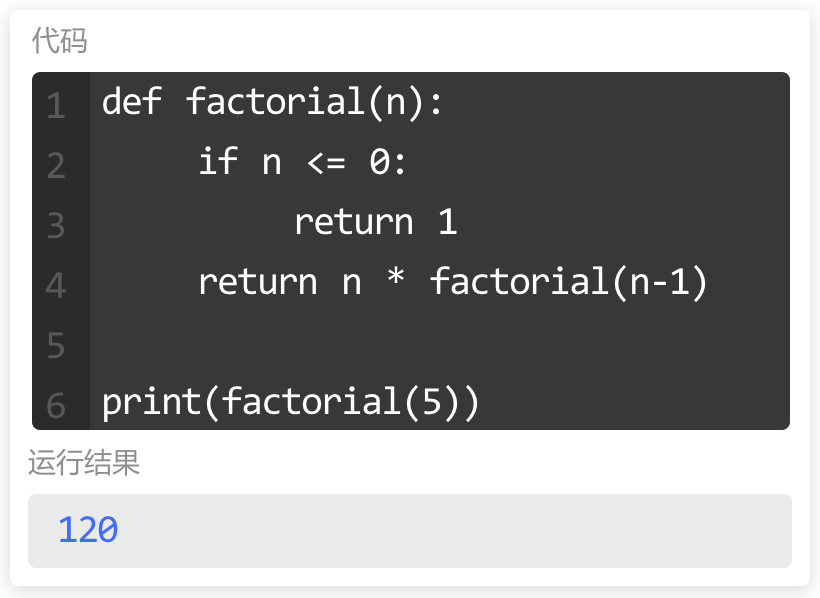### 小练习

200以内求和

``````# 定义名为sum()函数，传入参数n
def sum(n):
# 如果n小于等于0
if n <= 0:
# 返回0
return 0
# 返回n加上sum(n-1)
return n + sum(n-1)

# 调用sum()传入200并输出
print(sum(200))``````
• 0人Love
• 0人Haha
• 0人Wow
• 0人Sad
• 0人Angry
Python、函数、匿名函数、递归